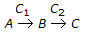# Chemical Engineering - Chemical Reaction Engineering

Exercise : Chemical Reaction Engineering - Section 5
1.
An isothermal aqueous phase reversible reaction, PR, is to be carried out in a mixed flow reactor. The reaction rate in k.mole/m3 .h is given by, r = 0.5CP - 0.125CR. A stream containing only P enters the reactor. The residence time required (in hours) for 40% conversion of P is
0.80
1.33
1.60
2.67
Explanation:
No answer description is available. Let's discuss.

2.
Design of heterogeous catalytic reactor involves consideration of __________ steps.
only chemical
only physical
both (a) & (b)
neither (a) nor (b)
Explanation:
No answer description is available. Let's discuss.

3.
A consecutive reaction,, is characterised by
maxima in the concentration of A.
maxima in the concentration of B.
maxima in the concentration of C.
high exothermicity.
Explanation:
No answer description is available. Let's discuss.

4.
What is the unit of the rate constant in a chemical reaction in which 10% of the reactant decomposes in one hour, 20% in two hours, 30% in three hours and so on ?
Litre/mole.second
Moles/litre.second
Litre/mole
Litre/second